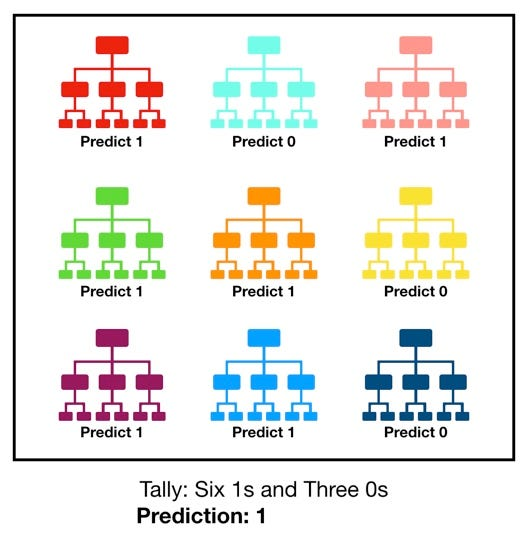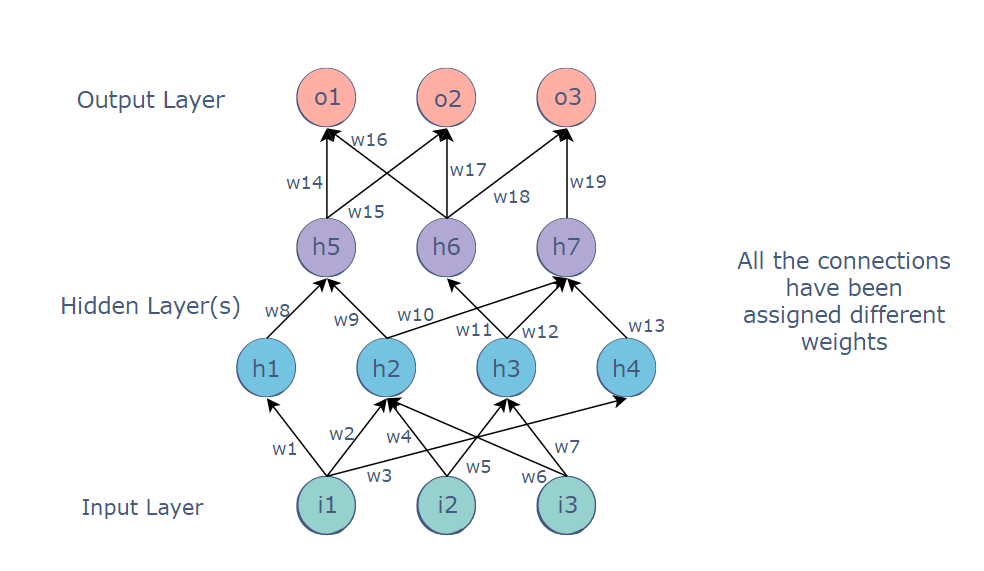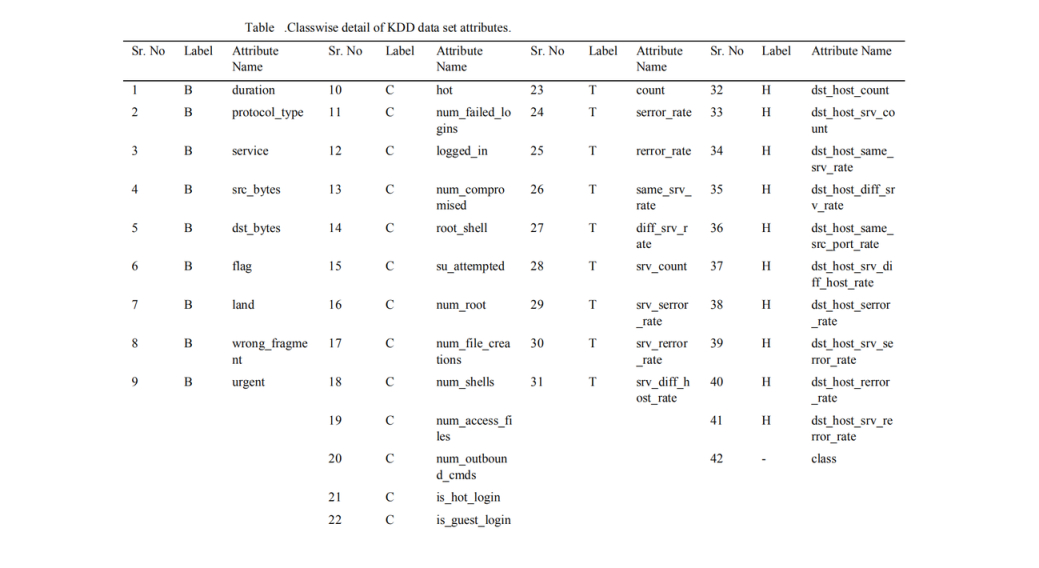# Anomaly based Intrusion Detection System

Intrusion Detection is the art of sensing when a system or network is being used inappropriately or without authorization. Without intrusion detection, an attacker can attempt attacks many times until an attack is finally successful. Intrusion detection allows for these attacks to be identified before a successful attack is likely to occur.
In this blog, we’ll talk about an Anomaly detection based approach for Intrusion Detection System. First of all, let’s discuss what the whole concept of anomaly based intrusion detection is.

Anomaly based intrusion detection uses statistics to form a baseline usage of the networks at different time intervals. This system uses machine learning to create a model simulating regular activity and then compares new behaviour with the existing model.

Before we continue with defining anomaly based IDS, here are a few things you should know:

• Machine Learning is the field of study that gives computers the capability to learn and improve from experience without being programmed to do so explicitly.

• The process of learning begins with observations or data to look for patterns in data and make better predictions based on the dataset provided. The primary objective is to allow computers to learn without human assistance and adjust its actions accordingly.

Machine Learning Algorithms can be broadly classified into:

• Supervised machine learning algorithms: It is called supervised learning because the process of an algorithm learning from the training dataset can be thought of as a teacher supervising the learning process. We know the correct answers, the algorithm iteratively makes predictions on the training data and is corrected by the teacher. Learning stops when the algorithm achieves an acceptable level of performance. The teacher here is the labelled dataset, that provides us with information & facilitates learning.

• Unsupervised machine learning algorithms: are used when the information used to train is neither labelled nor classified. Unattended learning studies how systems can infer a function to describe a hidden structure from unlabeled data.

• Semi-supervised machine learning algorithms: makes use of unlabeled data for training — with a blend of less labelled data and a lot of unlabeled data. Semi-supervised learning falls between unsupervised learning and supervised learning

## Evaluation of different Machine Learning Models for IDS

Unsupervised learning algorithms can learn the typical pattern of the network and can report outliers without labelling the dataset. It can detect new types of intrusions but is extremely prone to false positives. To reduce the false positives, we can introduce a labelled dataset and build a supervised machine learning model by teaching it the difference between a normal and an attack packet in the network, basically we will be labelling the normal & malicious traffic. The supervised model can handle the known attacks deftly and can also recognise variations of those attacks. Standard supervised algorithms such as Naive Bayes, Random Forest Classifier, MLP and Deep Neural Network are discussed below.

Apart from the algorithm used, one of the most important things while writing a Machine model and training it, is the dataset used. And the quality and extensiveness of the dataset determines the quality of the model & how well it will perform generally. For many years, KDD99 Dataset has been a benchmark for IDS model training & evaluations. But to fully customise your model as per your network server and to train your model to handle anomalies in your network, it is important for it to train a model specifically for your network, and let the model learn what normal traffic looks like for your network i.e. normal and anomalous traffic for each network is different. One can use both supervised and unsupervised learning for this purpose.

To solve this problem & generate customisable dataset, we have implemented Network Intrusion Dataset Generator that lets you capture your network traffic using scapy and generates a csv file that can readily be used for training your model after preprocessing the dataset. The best part is, it is fully customizable and one can choose to keep whatever features they want. You can find the implementation here

Code presented here is an implementation to generate csv file from packets captured on the network. One can also convert already captured packets in a pcap file to csv. The current implementation hasn’t applied many filters on capturing of packets & can be extended according to one’s own need.

Coming back to different models, let’s evaluate how each model fares on KDD99 Dataset (for testing we’ve used KDD99 Dataset). We have used scikit-learn models for the evaluation, implementation of all the models can be found in this colab notebook here.

Let’s talk in brief about what each model does (we won’t discuss which model is the best here since each model will serve good for different purposes & different datasets, so for now we’ll skip discussing the metrics. Although, if one wishes they can look at the accuracy we are getting for the KDD99 dataset for each model by running the cells in the colab notebook.

### Random Forest Classifier :

Random forest, like its name implies, consists of a large number of individual decision trees that operate as an ensemble. Each individual tree in the random forest spits out a class prediction and the class with the most votes becomes our model’s prediction It basically is like an election where uncorrelated models votes to choose a class, and the rule is majority wins.Figure 1. Visualization of how Random Forest Classifier works

### Naive Bayes Classifier :

Naive Bayes classifiers are a collection of classification algorithms based on Bayes’ Theorem. It is not a single algorithm but a family of algorithms where all of them share a common principle, i.e. every pair of features being classified is independent of each other.

### MLP (Multi-layered Perceptron) :

A multi-layered perceptron consists of interconnected neurons transferring information to each other, much like the human brain. Each neuron is assigned a value. The network can be divided into three main layers :

• Input Layer

• Hidden Layer(s)

• Output LayerFigure 2. Representation of Multi-layered Perceptron

The MLP is a feedforward neural network, which means that the data is transmitted from the input layer to the output layer in the forward direction. The connections between the layers are assigned weights. These weights are calculated by a mathematical function and adjusted based on the provided dataset to minimize the cost function.

### Deep Neural Network

Deep Neural Network is similar to MLP, it’s just that it supports backpropagation. Backpropagation is a technique used to optimize the weights of a neural net using the outputs as inputs. Conventionally, random weights are assigned to all the connections. These random weights propagate values through the network to produce the output. Naturally, this output would differ from the expected output. The difference between the two values is called the error.
Backpropagation refers to the process of sending this error back through the network, readjusting the weights automatically so that eventually, the error between the actual and expected output is minimized.

### Feature Analysis

Enough on briefing each separate model, let’s have a look at the feature analysis of KDD dataset. This brief discussion below is based on research paper.

The KDD data set has 42 attributes. The attribute labeled 42 in the data set is the ‘class’ attribute which indicates whether a given instance is a normal connection instance or an attack. Out of these 42 attributes, 41 attributes can be classified into four different classes as discussed below:

• Basic, B Features are the attributes of individual TCP connections

• Content ,C features are the attributes within a connection suggested by the domain knowledge

• Traffic, T features are the attributes computed using a two-second time window

• Host, H features are the attributes designed to assess attacks which last for more than two secondsFigure 3. Attributes in KDD99 Dataset

The conclusion of the result is that Basic features alone results in highest accuracy. One can find more details and all the metrics in the research paper provided above.

## Summary:

In this blog, we introduced an anomaly based Intrusion Detection System & discussed various machine learning models & its implementation.